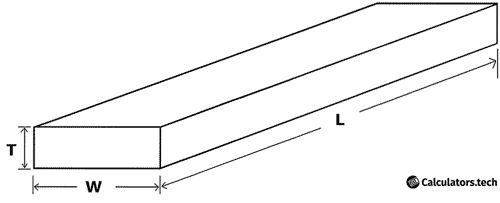# board foot calculator

Enter Information

#### RESULTS

Fill the calculator form and click on Calculate button to get result here

Get board foot calculator For Your Website

## What is Board Foot?

The term “board foot” has a simple and easy to understand the definition. Woodcutters and carpenters purchase logs with individual volumes. A board foot is used to determine the volume of these logs. It can be converted easily to cubic inches. For conversion purposes, the following scale is used

$$144 \mathbf{\small{inches}} = 1 \mathbf{\small{Board Foot}}$$

Thus, if you wish to convert 2 Board Foot, it would be

$$2 \times144 = 288 \small {\mathbf{cubic inches}}$$

## How to Calculate Board Foot?

To calculate board foot for a collection of logs, you should be well aware of the formula.

### Board Foot Formula:

To calculate board foot, you need to use the formula given below

Board Feet = $$\dfrac{L \times W \times T }{12}$$

The parameters used above have the following interpretations

• L is the length of the log (length is in ft)
• W is the width of the log (width is in inches)
• T is the thickness of the log (thickness is in inches)Consider that you have a log with length 12 ft, width 13 inches and thickness 10 inches. Thus, board would be given as

Board Feet = $$\dfrac{12 \times 13 \times 10 }{12}$$

Board Feet = $$130 \mathbf{\small{BF}}$$

## Determination of Total Cost (Lumber Calculator)

If you are well aware of the Board Feet formula, it would be convenient to determine the cost of multiple logs purchased.

## Selection of Number of Logs and confirming dimensions

Before advancing to the stage of calculation, one has to be sure about the number of log pieces that need to be procured. Along with that, the dimensions of each piece have to be confirmed. Consider that you have to procure 8 log pieces and each one has the following dimensions.

L = 15 ft
W = 18 in
T = 14 in.

Here are the steps you need to complete

### 1.    Determine the Board Feet of one Log

To start with, we need to determine the board feet for one log. Let us insert the values in the formula given below.

Board Feet = $$\dfrac{L \times W \times T }{12}$$

Board Feet = $$\dfrac{15 \times 18 \times 14 }{12}$$

Board Feet = $$315 \mathbf{\small{BF}}$$

The above is the value for one log. Once this value has been determined, the total board feet according to the number of log pieces to be purchased has to be determined.

### 2.    Multiply the individual value with the number of log pieces being purchased

In accordance with the scenario mentioned above, a total of 8 log pieces have to be purchased. Hence, multiple the value for one log by 8.

Board Feet for 8 pieces = $$315 \times 8$$

Board Feet for 8 pieces = $$2520 \mathbf{\small{BF}}$$

### 3.    Calculating the Cost

Consider that the rate for one BF is $$\15$$. According to the above results, the total value for 8 pieces is $$2520 \mathbf{\small{BF}}$$. Hence, total cost would be calculated as follows.

Total Cost = $$\mathrm{Total BF \times Cost of one BF}$$

Total Cost = $$2520 \times 15$$

Total Cost = $$\37800$$

Other Languages
##### Not Available for board foot calculator
Related Calculators